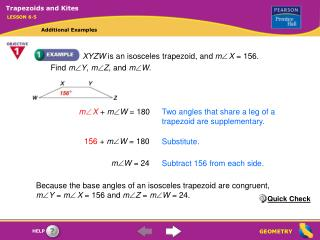# XYZW is an isosceles trapezoid, and m  X = 156. Find m  Y , m  Z , and m  W . - PowerPoint PPT PresentationDownload PresentationXYZW is an isosceles trapezoid, and m  X = 156. Find m  Y , m  Z , and m  W .

XYZW is an isosceles trapezoid, and m  X = 156. Find m  Y , m  Z , and m  W .Download Presentation## XYZW is an isosceles trapezoid, and m  X = 156. Find m  Y , m  Z , and m  W .

- - - - - - - - - - - - - - - - - - - - - - - - - - - E N D - - - - - - - - - - - - - - - - - - - - - - - - - - -
##### Presentation Transcript

1. mX + mW = 180 Two angles that share a leg of a trapezoid are supplementary. 156 + mW = 180 Substitute. mW = 24 Subtract 156 from each side. Trapezoids and Kites LESSON 6-5 Additional Examples XYZW is an isosceles trapezoid, and mX = 156. Find mY, mZ, and mW. Because the base angles of an isosceles trapezoid are congruent, mY = mX = 156 and mZ = mW = 24. Quick Check

2. Trapezoid ABDC is part of an isosceles triangle whose vertex angle is at the center of the spider web. Because there are 6 adjacent congruent vertex angles at the center of the web, together forming a straight angle, each vertex angle measures , or 30. 180 6 Trapezoids and Kites LESSON 6-5 Additional Examples Half of a spider’s web is shown below, formed by layers of congruent isosceles trapezoids. Find the measures of the angles in ABDC. By the Triangle Angle-Sum Theorem, mA + mB + 30 = 180, so mA + mB = 150. Because ABDC is part of an isosceles triangle, mA = mB, so 2mA = 150 and mA = mB = 75.

3. Another way to find the measure of each acute angle is to divide the difference of 180 and the measure of the vertex angle by 2: 180 – 30 2 = 75 Because the bases of a trapezoid are parallel, the two angles that share a leg are supplementary, so m C = m D = 180 – 75 = 105. Trapezoids and Kites LESSON 6-5 Additional Examples (continued) Quick Check

4. Diagonals of a kite are perpendicular. m 2 = 90 RU = RS Definition of a kite m 1 = 72 Isosceles Triangle Theorem m 3 + mRDU + 72 = 180 Triangle Angle–Sum Theorem m RDU = 90 Diagonals of a kite are perpendicular. m 3 + 90 + 72 = 180 Substitute. m 3 + 162 = 180 Simplify. m 3 = 18 Subtract 162 from each side. Trapezoids and Kites LESSON 6-5 Additional Examples Find m 1, m 2, and m 3 in the kite. Quick Check